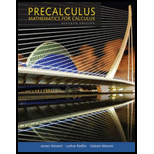Chapter 5, Problem 10RCC### Precalculus: Mathematics for Calcu...

7th Edition
James Stewart + 2 others
ISBN: 9781305071759

#### Solutions

Chapter
Section### Precalculus: Mathematics for Calcu...

7th Edition
James Stewart + 2 others
ISBN: 9781305071759
Textbook Problem

# (a) Define the inverse sine function, the inverse cosine function, and the inverse tangent function. (b) Find sin − 1 1 2 , cos − 1 2 2 , and tan−1 1. (c) For what values of x is the equation sin(sin−1 x) = x true? For what values of x is the equation sin−1(sin x) = x true?

(a)

To determine

To define: The inverse sine function, inverse cosine function and tangent function.

Explanation

The inverse sine function is defined as the function which is the reciprocal of sine function.

The inverse sine function is the function of sin1 with the domain [1,1] and range [π2,π2] is defined by,

sin1x=ysiny=x

The sine inverse function is also called as arcsine and denoted by arc sin.

The inverse cosine function is defined as the function which is the reciprocal of cosine function.

The inverse cosine function is the function of cos1 with the domain [1,1]

(b)

To determine

To evaluate: The value of inverse of sin112 , cos122 , and tan11 .

(c)

To determine

To evaluate: The value of x for which the inverse sine function is true.

### Still sussing out bartleby?

Check out a sample textbook solution.

See a sample solution

#### The Solution to Your Study Problems

Bartleby provides explanations to thousands of textbook problems written by our experts, many with advanced degrees!

Get Started

## Additional Math Solutions

#### Find more solutions based on key concepts